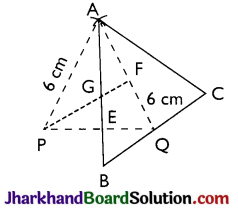# JAC Class 9 Maths Important Questions Chapter 11 Constructions

Jharkhand Board JAC Class 9 Maths Important Questions Chapter 11 Constructions Important Questions and Answers.

## JAC Board Class 9th Maths Important Questions Chapter 11 Constructions

Question 1.
Construct an equilateral triangle if its altitude is 3.2 cm.
Solution :
Given: In an equilateral ΔABC, an altitude
Required: To Construct an equilateral triangle ABC from the given data.
STEPS:
(i) Draw a line PQ
(ii) Construct a perpendicular bisector DE to PO.
(iii) Cut off DA = 3.2 cm from DE.
(iv) Construct ∠DAR = 30°.
The ray AR intersects PQ at B.
(v) Similarly, draw ∠DAC = 30.
The ray AC intersects PQ at C.
(vi) Join A with B and C.
We get the required ΔABC.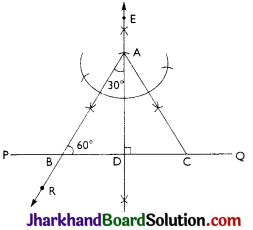Question 2.
Construct a right-angled triangle whose hypotenuse measures 8 cm and one side is 6 cm.
Solution :
Given: Hypotenuse AC of a ΔABC = 8 cm and one side AB = 6 cm.
Required: To construct a right-angled ΔABC from the given data.
STEPS:
(i) Draw a line segment AC = 8 cm.
(ii) Mark the mid-point 0 of AC by doing perpendicular bisector of AC.
(iii) With O as centre and radius OA, draw a semicircle on AC.
(iv) With A as centre and radius equal to 6 cm, draw an arc, cutting the semicircle a B.
(v) Join A and B, B and C.
We get the required right-angled triangle ABC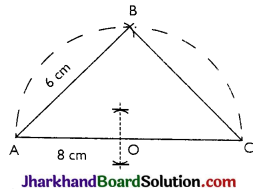Question 3.
Construct a ΔABC in which BC = 6.4 cm, altitude from A is 3.2 cm and the median bisecting BC is 4 cm.
Solution :
Given: One side BC = 6.4 cm, altitude AD = 3.2 cm and the median AL = 4 cm.
Required: To construct a ΔABC form the given data
STEPS:
(i) Draw BC = 6.4 cm
(ii) Bisect BC at L.
(iii) Draw EF || BC at a distance 3.2 cm for BC
(iv) With L as centre and radius equal to 4 cm, draw an arc, cutting EF at A
(v) Join A and B ; A and C, A and L.
We get the required triangle ABC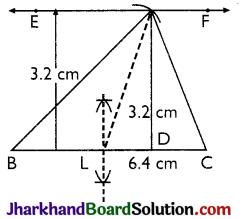Question 4.
Construct a ΔABC in which ∠B = 30° and ∠C = 60° and the perpendicular from the vertex A to the base BC is 4.8 cm.
Solution :
Given: ∠B = 30°, ∠C = 60°, length of perpendicular from vertex A to be base BC = 4.8 cm.
Required: To construct a ΔABC from the given data.
STEPS :
(i) Draw any line PQ.
(ii) Take a point B on line PQ and construct ∠QBR = 30°
(iii) Draw a line EF || PQ at a distance of 4.8 cm from PQ, cutting BR at A.
(iv) Construct an angle ∠FAC = 60°, cutting PQ at C.
(v) Join A and C.
We get the required triangle ABC.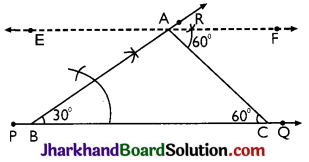Question 5.
Construct a triangle ABC, the lengths of whose medians are 6 cm, 7 cm and 6 cm.
Solution :
Given: Median AD = 6 cm, median BE = 7 cm, median CF = 6 cm.
Required: To construct a AABC from the given data.
STEPS:
(i) Construct a ΔAPQ with AP = 6 cm, PQ = 7 cm and AQ = 6 cm.
(ii) Draw the medians AE and PF of ΔAPQ intersecting each other at G.
(iii) Produce AE to B such that GE = EB
(iv) Join B and Q and produce it to C, such that BQ = QC
(v) Join A and C. We get the required triangle ABC.